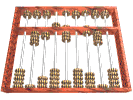# Mrs. Ellerson's 4th Grade Math ClassThe students will practice many fourth grade math skills this year. Learning mathematics results more than a mastery of basic skills. In my mathematical classroom I will help students develop mathematical understanding, learn important facts, skills, and procedures and also help them develp the ability to apply the processes of mathematics in hopes of helping students acquire a positive attitude towards mathematics.Learning mathematics results in more than a mastery of basic skills. Students will also develop mathematical understanding and problem solving skills. Problem solving and understanding math vocabulary have become an integral part of our math program. Many times, students will have to read through and interpret information before solving the math problem. Mathematics is a powerful learning tool.  Students will develop the ability to use mathematics to extend and apply their knowledge.